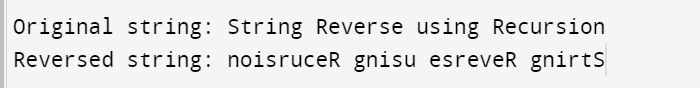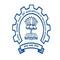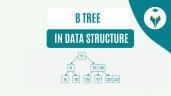Coding Interview Question

# Coding Interview Question

clickHere
Updated on Oct 3, 2023 11:35 IST

For any technical job interview, there must be a technical interview round. In that round you will be asked questions related to different data structure and algorithm, and your coding skill will be tested. In this article, we will discuss 20+ coding interview question.

Coding Interview is an integral part of the interview process for technical job profile in any IT company. You will be given some set of coding questions, to check your coding skills and you have to solve them depending on the programming language you know. It can be C, C++, Python, Java, HTML, JavaScript, SQL or else. In this article, we will discuss more than 20+ coding interview questions, that are most likely to be askes in your interview rounds.

## Theoretical Questions

#### 1. What is a Data Structure?

Data Structure is a way to demonstrate, organize, process, and manipulate the data in a system. It shows the relationship between data elements and enhances efficiency, reusability, and abstraction.

#### 2. What is an Array?

An array is a linearly-ordered data structure that stores similar data elements at a contiguous memory location.

Arrays are of two types:

• Single-Dimensional Array
• Multi-Dimensional Array

Also Explore: Implementing Array in Java

Also Explore: Implementing Array in C

#### 3. What is Linked List?

A Linked List is used to collect data in the form of nodes, and each node stores two elements- data and the address of the next node.

Linked List are of two types:

Also Explore: Introduction to Linked List in Data Structure

Also Explore: Linked List Examples in C and Python

#### 4. What is Stack?

Stack is an ordered collection of items that work on the principle of LIFO (Last in First Out), i.e., the item stored in the last will come out first.

It uses the following Operations:

• pop()
• Push(item)
• Peek()
• isEmpty()

Also Explore: All that you need to know about Stack

#### 5. What is Queue?

Queue stores the data sequentially. It uses FIFO (First-in-First-Out) approach for accessing elements i.e.; items are removed from the data structure in the order they got added.

It uses the following Operations:

• Remove()
• Peek()
• IsEmpty()

#### 6. What is Hash Table?

It maps the key to a value pair for high-efficient lookup using hash functions. The hash function computes an index into an array in which elements are stored.

Two common ways to implement Hash Table:

• an array of linked lists and a hash code function to insert a key and value
• hash tables with a balanced binary search tree which gives O(log N) lookup time

Also Explore: Memory Management in Python

#### 7. What is Heap?

It is a special case of binary trees in which the parent nodes are compared to their child nodes with their value and arranged values.

There are two types of Heap:

• Min Heap
• Max Heap

Also Explore: All About Heap Sort Technique

#### 8. What is Tree in Data Structure?

A tree is a non-linear hierarchical data structure that consists of nodes connected by edges, and each node stores a value and list of references of the other nodes.

• Each tree has a root node on the top
• Each node can have zero or more child node

Binary Tree: A binary tree is an ordered tree where each node has a maximum of two children. These are referred to as the Left and Right Child.

Also ExploreTypes of Binary Trees in Data Structure

#### 9. What is Graph?

A graph is a non-linear data structure with a set of nodes (vertices) connected by edges. Data are stored in the nodes, and relationships are expressed using edges.

• Edges may be directed (with direction) or undirected (without direction)

#### 10. What is Recursion?

Recursion is the process of repeating itself in a self-similar way, i.e., a technique to solve a complex computer problem by creating a function that calls itself to work on a more minor problem.

Also Explore: Introduction to Recursion Function in Python

#### 11. What is a String?

A string is a data type in programming used to represent the text rather than a number. A sequence of characters contains symbols, letters, numbers, or spaces.

• Characters must be enclosed in the quotations.

Also Explore: Strings in Python

#### 12. What are the OOPs Concepts?

OOPs (Object-Oriented Programming) refers to the language that uses objects in programming. It is used for designing programs using classes and objects. It provides concepts such as inheritanceabstractionpolymorphismencapsulation, etc.

Also Explore our Web Story: OOPs with AnalogyUnderstanding OOPs Concepts in Java
Object oriented programming (OOP) concepts are the fundamental pillars of programming. The article below explains the OOPs concept in Java. It covers introductions to OOPs, class, objects, inheritance, abstraction, encapsulation,...read moreOOPs Concepts in C++
OOP concepts in C++ involve creating objects from classes, bundling data and methods. Inheritance forms new classes from existing ones, while polymorphism enables interchangeable usage of objects, enhancing code modularity...read moreObject-Oriented Programming with Python
A good understanding of OOPs concepts can help you make your Python programming journey smoother. In this article, we will explore the basics of object-oriented programming in Python.

#### 13. What is Bubble Sort Algorithm?

Bubble Sort is one of the simplest sorting algorithms that compare adjacent elements and swap them if they are lesser/ greater based on the conditions mentioned i.e., each element is compared with all the other elements in the array till the final element is found.

Also Explore: Bubble Sort Algorithm (with Codes)

## Coding Questions

#### 1. Reverse a String

There are different ways to reverse a string; here, we will show using recursion in Java.

` `
`class Reverse_String_Recursion{   static void Reverse(String str)   {       if ((str==null)||(str.length() <= 1))           System.out.println(str);       else       {           System.out.print(str.charAt(str.length()-1));           Reverse(str.substring(0,str.length()-1));       }   }    public static void main(String[] args) {       String str1  =  "String Reverse using Recursion";       System.out.println("Original string: "+str1);       System.out.print("Reversed string: ");        // recursive function call to Reverse()       Reverse(str1);   }}Copy code`

OutputAlso Check: Reverse a String in Java

Also Check: Reverse a String in Python

#### 2. Armstrong Number

` `
`#Enter inputnum = int(input("Enter 3-digit number : ")) sum = 0temp = num#Define a functionwhile temp > 0:    digit = temp % 10    sum += digit * digit * digit    temp = temp//10 if sum==num:    print('It is an Armstrong number')else:    print('It is not an Armstrong number)Copy code`

Also Check: How to Find Armstrong Number using Python

#### 3. Palindrome Words

` `
`#Define a function def isPalindrome(string):     if (string == string[::-1]) :         return "The string is a palindrome."     else:         return "The string is not a palindrome."  #Enter input string string = input ("Enter string: ")  print(isPalindrome(string))Copy code`

#### 4. Prime Number

` `
`#Prime numbers are always greater than 1 if num > 1:     #Check for factors     for i in range(2, num):         if (num % i) == 0:             #If factor is found, set flag to True             flag = True             #Break out of loop             break  #Check if flag is True if flag:     print(num, "is not a prime number") else:     print(num, "is a prime number")Copy code`

Also Check: What is a Prime Number?

#### 5. Fibonacci Number

` `
`#Generate the Fibonacci number using recursion#define a recursive function def feb(n):    if n == 0:        return 0  #first fibonacci number F_0 = 0    elif n == 1:        return 1  #second fibonacci number F_1 = 0    else:        return (feb(n - 1) + feb(n - 2))  #every fibonacci number is sum of previous two n = int(input("Enter the number"))  #enter the number of terms neededfor i in range(0, n):    print (feb(i))Copy code`

Also Check: Fibonacci Number in Python, C, Java

#### 6. Leap Year

` `
```def checkleapyear(y): return(((y % 4 == 0) and (y % 100 != 0)) or (y % 400 ==0)); #The above line Returns true if the year is a multiple of 4 and not a multiple of 100 OR the year is a multiple of 400 as mentioned per the syntax logic above. y= 2000 if (checkleapyear(y)):print("The given year is leap year") else: print("The given year is not a leap year")Copy code```

Also Check: Python Program to Check Leap Year

#### 7. Calculate Variance

` `
`import statistics # Creating a sample of datasample = [2.74, 1.23, 2.63, 2.22, 3, 1.98] print("Variance of sample set is % s"      %(statistics.variance(sample)))Copy code`

Also Check: Measure of Dispersion

#### 8. Factorial of a number

` `
`#include int main(){    int n,i,fact;    fact = i = 1;    printf("Enter a Positive Number: ");    scanf("%d",&n);    do    {        fact = fact * i;        i++;    }while(i<=n);    printf("The Factorial of %d is : %d",n,fact);    return 0;}Copy code`

Also Check: What is Factorial of a Number?

Also Check: How to find Factorial of a Number in Python

#### 9. Merge Two Sorted List

` `
`public class Solution {    public ListNode mergeTwoLists(ListNode l1, ListNode l2) {        ListNode fakeNode = new ListNode(-1);        ListNode node = fakeNode;        while (l1 != null && l2 != null){            if (l1.val < l2.val){                node.next = l1;                l1 = l1.next;                node = node.next;            }//if            else{                node.next = l2;                l2 = l2.next;                node = node.next;             }//else        }//while l1 & l2        if (l1 != null){            node.next = l1;        }        else if (l2 != null){            node.next = l2;        }        return fakeNode.next;    }}Copy code`

Also Check: Merge Sort Algorithm

#### 10. Swap two number without using third variable

` `
`#includeint main(){    int a, b;    printf("Enter two numbers: ");    scanf("%d %d", &a, &b);  //consider two numbers as 4 and 5    a = a + b;  //a = 4 + 5 = 9    b = a - b;  //b = 9 - 5 = 4    a = a - b;  //a = 9 - 4 = 5    printf("Numbers after swapping: %d %d", a, b);}Copy code`

## Conclusion

In this article, we have briefly discussed more than 20+ coding interview question.

Hope you will like the article.

Keep Learning!!

Keep Sharing!!

clickHere

Vikram has a Postgraduate degree in Applied Mathematics, with a keen interest in Data Science and Machine Learning. He has experience of 2+ years in content creation in Mathematics, Statistics, Data Science, and Mac... Read Full Bio

## Trending Technology CoursesHTML, CSS, and Javascript for Web Developers
Coursera4.4Python Training
IIT Bombay4.2Programming in Java by NPTEL
NPTEL4.6## Top Picks & New Arrivals